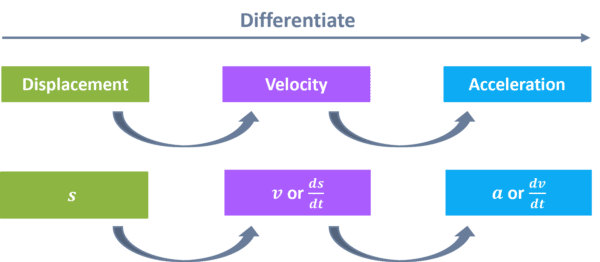# Differentiation with Linear Kinematics

GCSELevel 8-9Edexcel iGCSE

## Differentiation with Linear Kinematics

Kinematics looks at the motion of a particle, considering displacement, velocity, and acceleration. We can model the movement of anything as a particle, such as a car or a ball.

Make sure you are happy with the following topic before continuing:

## Displacement

Displacement is slightly different to distance…

We know distance is a measurement that is always positive – you cannot get a negative distance.

Displacement however, measures the distance from a fixed point of origin, and if this distance is forwards from the origin, the displacement is positive, and if it is backwards, the displacement is negative. We call displacement $s$, and it is usually measured in $\text{m}$.

For example, both the distance and displacement of the following ball from the origin is $4$:However, in the following example, while the distance is $3$, the displacement is $-3$:Level 8-9GCSEEdexcel iGCSE

## Velocity

Velocity measures a change of displacement, or how quickly the displacement is changing.

Velocity is usually written $v$, and measured in $\text{m/s}$. We can look at it in a similar way to displacement:

Velocity is slightly different to speed, as it also takes direction into consideration. If $v = 4$, then the particle is moving in the positive direction with a speed of $4 \text{m/s}$. If $v = -4$, then the particle is moving in the negative direction with a speed of $4 \text{m/s}$.

Level 8-9GCSEEdexcel iGCSE

## Acceleration

Acceleration measures a change of velocity, or how quickly the velocity is changing.

Acceleration is usually written $a$, and measured in $\text{m/s}^2$.

If $a = 3$, the particle is accelerating at $3\text{ m/s}^2$.

If $a = -3$, the particle is decelerating at $3\text{ m/s}^2$.

Level 8-9GCSEEdexcel iGCSE

## Using Differentiation

We can use differentiation to help us find displacement, velocity, and accelerationWe express displacement, velocity, and acceleration in terms of $t$, which is time, usually in seconds.

If we differentiate an expression for displacement, we would get an expression for velocity, and if we differentiate an expression for velocity, we would get an expression for acceleration.

Level 8-9GCSEEdexcel iGCSE

## Example 1: Using Differentiation

The displacement of a car is shown in the following expression:

$s = 6t^3 + 5t^2 + 11t -4$

Find an expression for the velocity and acceleration of this car.

[4 marks]

To find velocity, we differentiate once:

$s = 6t^3 + 5t^2 + 11t -4\\$

$\dfrac{\text{d}s}{\text{d}t} = 18t^2 + 10t + 11$

To find acceleration, we differentiate the velocity:

$v = 18t^2 + 10t + 11\\$

$\dfrac{\text{d}v}{\text{d}t} = 36t + 10$

Level 8-9GCSEEdexcel iGCSE

## Example 2: Applied Differentiation

A ball is moving along a straight line. The displacement of the particle, from fixed origin point $O$, at time, $t$ seconds is:

$s = 7t^2 - 5t - 12$

a) Find the particle’s initial distance from $O$

b) Find $t$ when the particle is at rest

[5 marks]

a) ‘Initial’ means $t=0$, so we can use $t=0$ in the displacement equation to find the initial distance:

$s = 7t^2 - 5t - 12\\$

$s = 7(0)^2 - 5(0) - 12\\$

$s = -12$

The initial displacement is $-12$, however, the question is asking for distance, so this is $12\text{ m}$.

b) When the particle is at rest, the velocity will be $0\text{ m/s}^2$, so we need to firstly differentiate to find the expression for velocity.

$s = 7t^2 - 5t - 12\\$

$\dfrac{\text{d}s}{\text{d}t} = 14t - 5\\$

Now we can sub in $\dfrac{ds}{dt}=0\text{ m/s}^2$

$0 = 14t - 5\\$

$14t = 5\\$

$t = \dfrac{5}{14}\text{ s}$

Level 8-9GCSEEdexcel iGCSE

## Differentiation with Linear Kinematics Example Questions

We need to differentiate the velocity to find the acceleration:

$v = 21t^2 + 5t - 11\\$

$\dfrac{\text{d}v}{\text{d}t} = 42t + 5$

Differentiate to find an expression for velocity:

$s = 7t^2 - 14t + 6\\$

$\dfrac{\text{d}s}{\text{d}t} = 14t - 14$

When $t = 2\text{ s}$, the velocity is:

$v = 14(2) - 14\\$

$v = 14\text{ m/s}$

Differentiate to find an expression for acceleration:

$v = 14t - 14\\$

$\dfrac{\text{d}v}{\text{d}t} = 14$

At $t=2\text{ s}$, the acceleration is $14\text{ m/s}^2$

Let’s find an expression for the acceleration:

$s = 3t^2 + 12t - 2\\$

$\dfrac{\text{d}s}{\text{d}t} = 6t+12\\$

$\dfrac{\text{d}v}{\text{d}t} = 6$

So, the acceleration is $6\text{ m/s}^2$.

As there is no $t$ in the equation for acceleration, we know it will not change if $t$ changes, and so will always remain constant at $6\text{ m/s}^2$.

## You May Also Like...### MME Learning Portal

Online exams, practice questions and revision videos for every GCSE level 9-1 topic! No fees, no trial period, just totally free access to the UK’s best GCSE maths revision platform.

£0.00